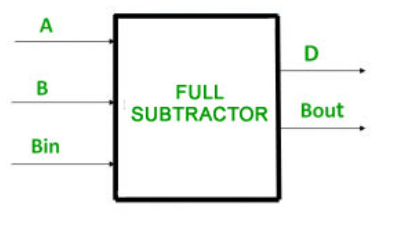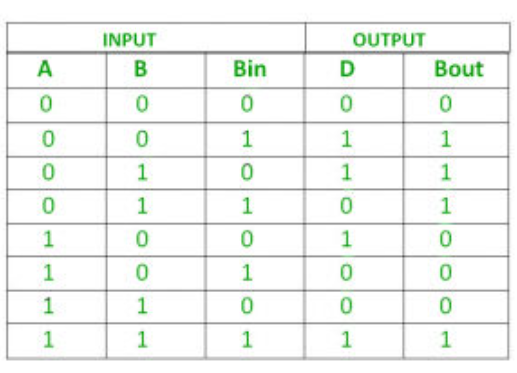# C++ program to implement full subtractor

Prerequisite : Full Subtractor
Given three inputs of Full Subtractor A, B, Bin. The task is to implement the Full Subtractor circuit and Print output states difference (D) and Bout of three inputs.

Introduction :
The full subtractor is used to subtract three 1-bit numbers  which are minuend, subtrahend, and borrow, respectively. The full subtractor has three input states and two output states. The two outputs are difference and borrow.Here we have three inputs A, B, Bin and two outputs D, Bout. And the truth table for full subtractor isLogical Expression :

```Difference = (A XOR B) XOR Bin
Borrow Out = Ā Bin + Ā B + B Bin```

Examples –

Input         :    0 0 1
Output      :     Difference=1, B-Out=1
Explanation :   According to logical expression Difference= (A XOR B) XOR Bin  i.e (0 XOR 0) XOR 1  =1 ,
B-Out=Ā Bin + Ā B + B Bin  i.e.  1AND 1 + 1 AND 0 + 0 AND 1 = 1.

Input         :    1 0 1
Output      :     Difference=0, B-Out=0

Approach :

• We will take three inputs A, B, Bin.
• By applying (A XOR B) XOR Bin gives the Value of Difference.
• By applying Ā Bin + Ā B + B Bin gives the value of B-Out.

## C++

 `//c++ code for above approach ` `#include ` `using` `namespace` `std; ` `//function to print difference and borrow out ` `void` `Full_Subtractor(``int` `A,``int` `B,``int` `Bin){ ` `  `  `   ``// Calculating value of Difference ` `    ``int` `Difference = (A ^ B) ^ Bin; ` `      `  `    ``//calculating NOT  value of a ` `    ``int` `A1 = not(A); ` `      `  `    ``//Calculating value of B-Out ` `    ``int` `B_Out = A1 & Bin | A1 & B | B & Bin; ` `  `  `    ``//printing the values ` `    ``cout<<``"Difference = "``<

Output

```Difference = 0
B-Out = 0
```
Whether you're preparing for your first job interview or aiming to upskill in this ever-evolving tech landscape, GeeksforGeeks Courses are your key to success. We provide top-quality content at affordable prices, all geared towards accelerating your growth in a time-bound manner. Join the millions we've already empowered, and we're here to do the same for you. Don't miss out - check it out now!

Previous
Next